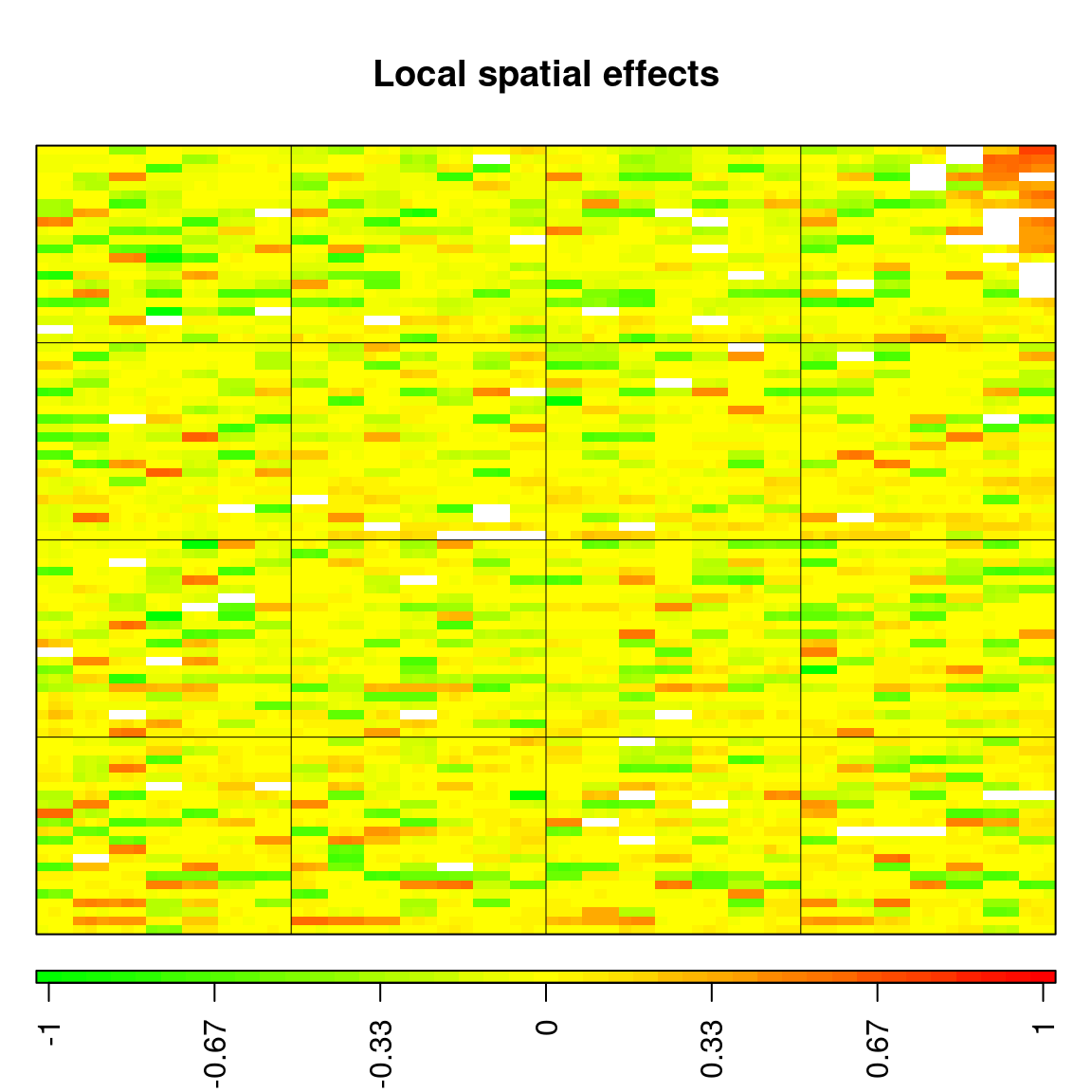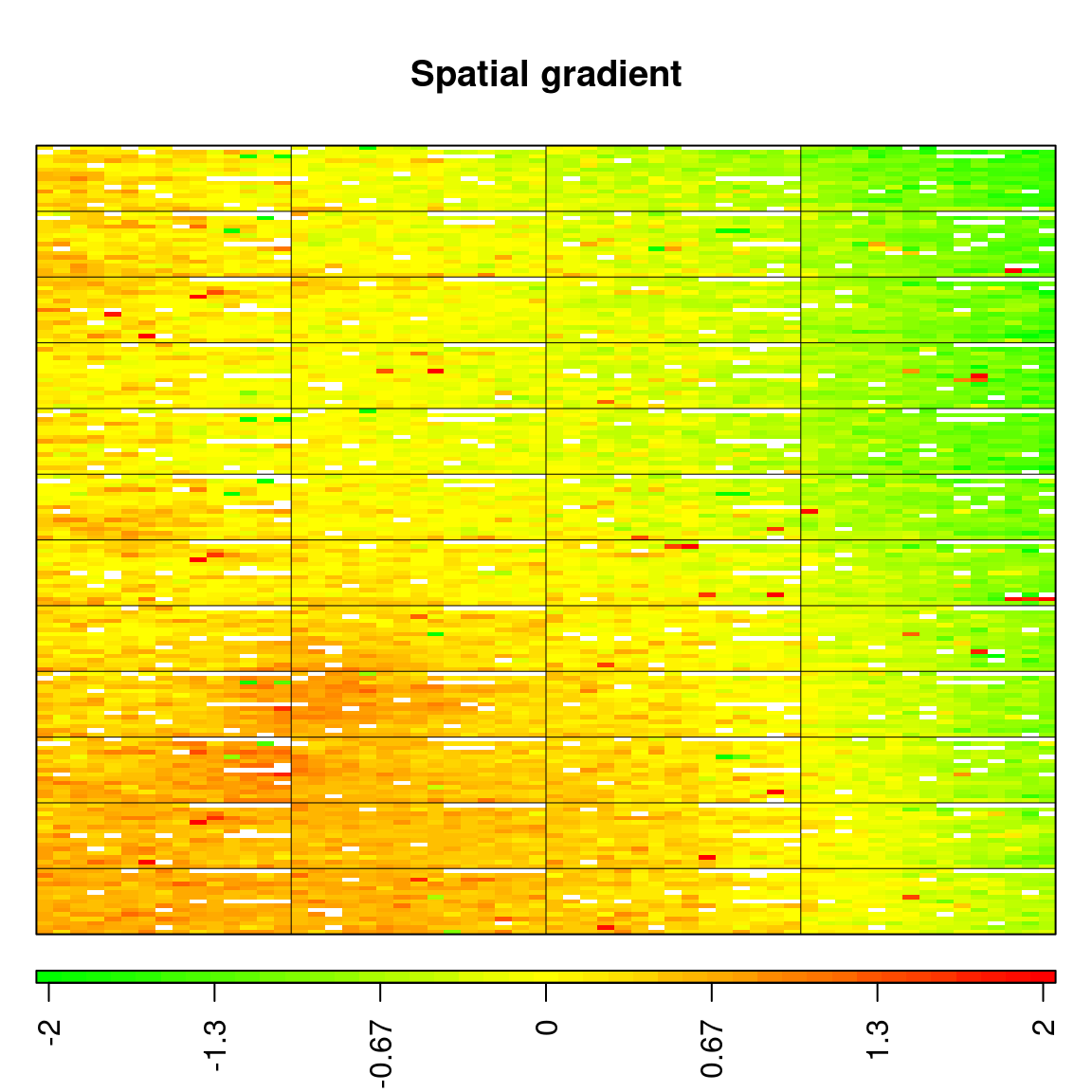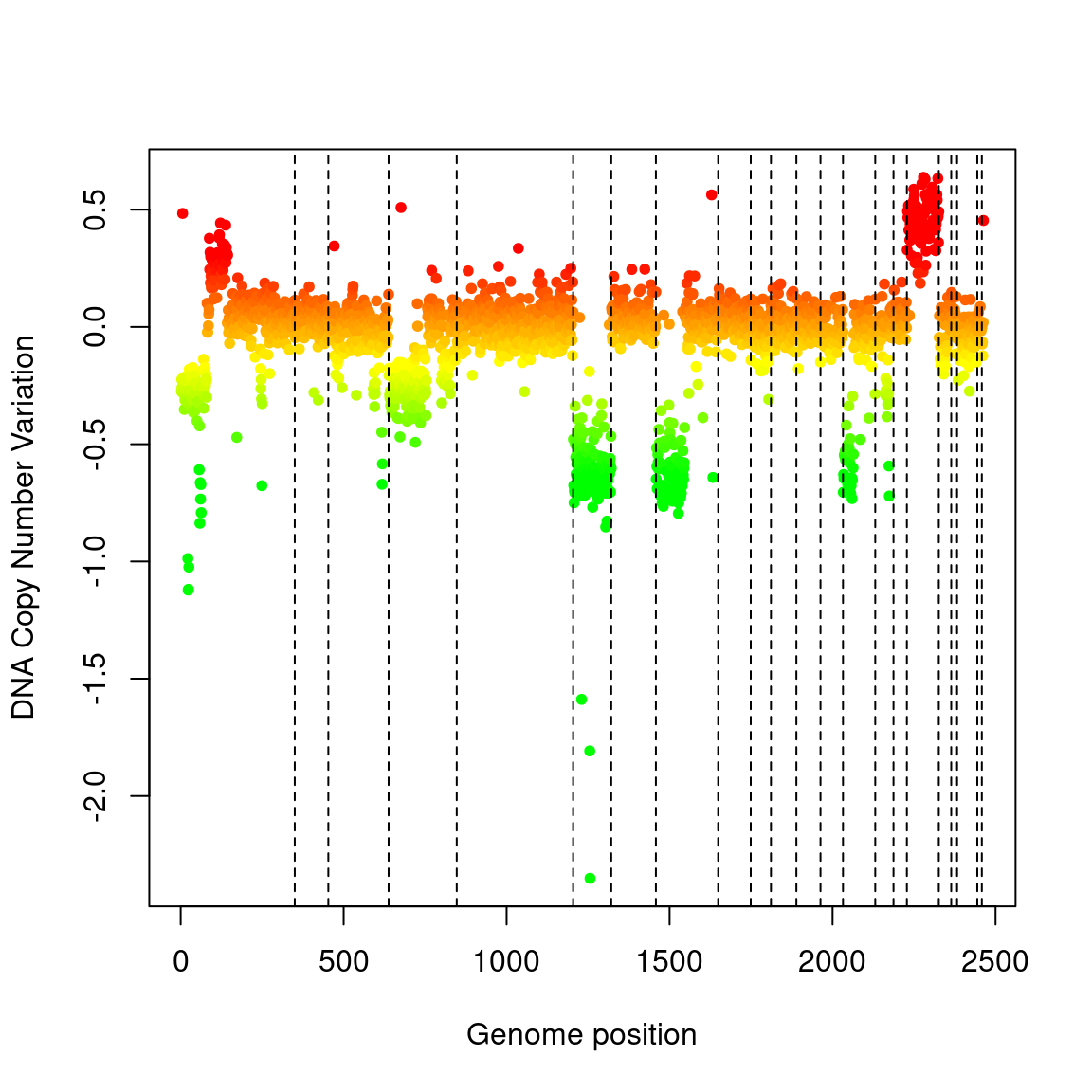1 Overview

This document gives an overview of the MANOR package, which is devoted to the normalization of Array Comparative Genomic Hybridization (array-CGH) data (Solinas-Toldo et al. 1997; Pinkel et al. 1998; Snijders et al. 2001; Ishkanian et al. 2004; Hupé et al. 2004). Normalization is a crucial step of microarray analysis which aims at separating biologically relevant signal from experimental artifacts. Typical input data is a file generated by an image analysis software such as GenePix or SPOT (Jain et al. 2002), containing several measurements for each biological variable of interest, i.e. several replicated spots for each clone; this spot-level data is filtered with various statistical criteria (including a spatial bias detection step which is described in (Neuvial et al. 2006)), and aggregated into clean clone-level data.

Using the arrayCGH framework developed in the package GLAD, which is available under Bioconductor. We propose the formalism of flags to handle clone and spot filtering: the core of the normalization process consists in applying to an arrayCGH object a list of flags that successively exclude from the data all irrelevant spots or clones.

We also define quality scores (qscores) that quantify the quality of an array after normalization: these scores can be used directly to compare the quality of different arrays after the same normalization process, or to compare the efficiency of different normalization processes on a given array or on a given batch of arrays.

This document is organized as follows: after a short description of optional items we add to arrayCGH objects (section arrayCGH class, we introduce the classes flag (section flag class) and qscore (section qscore class) with their attributes and dedicated methods; then we describe two useful graphical representation functions (section Graphical representations), namely genome.plot and report.plot; Afterwards we give a short description of the array-CGH datasets we provide (section Data); finally we illustrate the usage of MANOR by a sample R script (section Sample MANOR sessions).

1.1 Citing the MANOR package

##
## To cite package 'MANOR' in publications use:
##
##   Pierre Neuvial and Philippe Hupé (2021). MANOR: CGH Micro-Array
##   NORmalization. R package version 1.66.0.
##   http://bioinfo.curie.fr/projects/manor/index.html
##
## A BibTeX entry for LaTeX users is
##
##   @Manual{,
##     title = {MANOR: CGH Micro-Array NORmalization},
##     author = {Pierre Neuvial and Philippe Hupé},
##     year = {2021},
##     note = {R package version 1.66.0},
##     url = {http://bioinfo.curie.fr/projects/manor/index.html},
##   }
##
## ATTENTION: This citation information has been auto-generated from the
## package DESCRIPTION file and may need manual editing, see
## 'help("citation")'.

2arrayCGH class

For the purpose of normalization we have added several optional items to the arrayCGH objects defined in the R package GLAD, including:

• [cloneValues] a data frame with aggregated (clone-level) information, quite similar to profileCGH objects of GLAD
• [id.rep] the name of a variable common to cloneValues and arrayValues, that can be used as an identifier for the replicates.

3flag class

We view the process of filtering microarray data, and especially array-CGH data, as a succession of steps consisting in excluding from the data unreliable spots or clones (according to criteria such as signal to noise ratio or replicate consistency), and correcting signal values from various non-biologically relevant sources of variations (such as spotting effects, spatial effects, or intensity effects).

We introduce the formalism of flags to deal with this filtering issue: in the two following subsections, we describe the attributes and methods devoted to flag objects.

3.1flag attributes

A flag object f is a list whose most important items are a function (f\$FUN) which has to be applied to an object of class arrayCGH, and a character value (f\$char) which identifies flagged spots. Optionally further arguments can be passed to f\$FUN via f\$args, and a label can be added via f\$label. The examples of this subsection use the function to.flag, which is explained in subsection flag methods. 3.1.1 Exclusion and correction flags As stated above, we make the distinction between flags that exclude spots from further analysis and flags that correct signal values: 3.1.1.1 exclusion flags If f is an exclusion flag, f\$FUN returns a list of spots to exclude and f\$char is a non NULL value that quickly identifies the flag. In the following example, we define SNR.flag, a flag objects that excludes spots whose signal to noise ratio lower than the threshold snr.thr. SNR.FUN <- function(arrayCGH, var.FG, var.BG, snr.thr) { which(arrayCGH$arrayValues[[var.FG]] < arrayCGH$arrayValues[[var.BG]]*snr.thr) } SNR.char <- "B" SNR.label <- "Low signal to noise ratio" SNR.flag <- to.flag(SNR.FUN, SNR.char, args=alist(var.FG="REF_F_MEAN", var.BG="REF_B_MEAN", snr.thr=3)) 3.1.1.2 correction flags If f is a correction flag, f\$FUN returns an object of type arrayCGH and f\$char is NULL. In the following example, global.spatial.flag computes a spatial trend on the array, and corrects the signal log-ratios from this spatial trend: global.spatial.FUN <- function(arrayCGH, var) { if (!is.null(arrayCGH$arrayValues$Flag)) arrayCGH$arrayValues$LogRatio[which(arrayCGH$arrayValues$Flag!="")] <- NA ## Trend <- arrayTrend(arrayCGH, var, span=0.03, degree=1, iterations=3, family="symmetric") Trend <- arrayTrend(arrayCGH, var, span=0.03, degree=1, iterations=3) arrayCGH$arrayValues[[var]] <- Trend$arrayValues[[var]]-Trend$arrayValues$Trend arrayCGH } global.spatial.flag <- to.flag(global.spatial.FUN, args=alist(var="LogRatio")) 3.1.2 Permanent and temporary flags We introduce an additional distinction between permanent and temporary flags in order to deal with the case of spots or clone that are known to be biologically relevant, but that have not to be taken into account for the computation of a scaling normalization coefficient. For example in breast cancer, when the reference DNA comes from a male, we expect a gain of the X chromosome and a loss of the Y chromosome in the tumoral sample, and we do not want log-ratio values for X and Y chromosome to bias the estimation of a scaling normalization coefficient. Any flag object therefore contains an argument called type, which defaults to “perm” (permanent) but can be set to “temp” in the case of a temporary flag. In the following example, chromosome.flag is a temporary flag that identifies clones correcponding to X and Y chromosome: chromosome.FUN <- function(arrayCGH, var) { var.rep <- arrayCGH$id.rep
w <- which(!is.na(match(as.character(arrayCGH$cloneValues[[var]]), c("X", "Y")))) l <- arrayCGH$cloneValues[w, var.rep]

• a filtered array with field arrayCGH\$arrayValues\$Flag filled with the value of flag\$char for each spot to be excluded from further analysis in the case of an exclusion flag; • an array with corrected signal value in the case of a correction flag. args(flag.arrayCGH) ## function (flag, arrayCGH) ## NULL 3.2.3 flag.summary Function flag.summary computes spot-level information about normalization (including the number of flagged spots and numeric normalization parameters), and displays it in a convenient way. This function can either be applied to an object of type arrayCGH: args(flag.summary.arrayCGH) ## function (arrayCGH, flag.list, flag.var = "Flag", nflab = "not flagged", ## ...) ## NULL or to plain spot-level information, by using the default method: args(flag.summary.default) ## function (spot.flags, flag.list, nflab = "not flagged", ...) ## NULL 4qscore class As we point out in the introduction of this document, evaluating the quality of an array-CGH after normalization is of major importance, since it helps answering the following questions: - which is the best normalization process ? - which array is of best quality ? - what is the quality of a given array ? To this purpose we define quality scores (qscores), which attributes and methods are explianed in the two following subsections. 4.1qcsore attributes A qscore object qs is a list which contains a function (qs\$FUN), a name (qs\$name), and optionnally a label (qs\$label) and arguments to be passed to qs\$FUN (qs\$args). In the following example, the quality score pct.spot.qscore evaluates the percentage of spots that have passed the filtering steps of normalization; it provides an evaluation of the array quality for a given normalization process. The function to.qscore is explained in subsection qscore methods.

pct.spot.FUN <- function(arrayCGH, var) {
100*sum(!is.na(arrayCGH$arrayValues[[var]]))/dim(arrayCGH$arrayValues)
}
pct.spot.name <- "SPOT_PCT"
pct.spot.label <- "Proportion of spots after normalization"
pct.spot.qscore <- to.qscore(pct.spot.FUN, name=pct.spot.name, args=alist(var="LogRatioNorm"), label=pct.spot.label)

4.2qscore methods

4.2.1 to.qscore

The function to.qscore is used of the creation of qscore objects, with the specificities described in subsection [qscore attributes]

args(to.qscore)
## function (FUN, name = NULL, args = NULL, label = NULL, dec = 3)
## NULL

4.2.2 qscore.arrayCGH

Function qscore.arrayCGH simply computes and returns the value of qscore for arrayCGH:

args(qscore.arrayCGH)
## function (qscore, arrayCGH)
## NULL

4.2.3 qscore.summary.arrayCGH

Function qscore.summary.arrayCGH computes all quality scores of a list (using function qscore.arrayCGH), and displays the results in a convenient way.

args(qscore.summary.arrayCGH)
## function (arrayCGH, qscore.list)
## NULL

5 Data

We provide examples of array-CGH data coming from two different platforms. These data illustrate the need for appropriate within-array normalization methods, and especially the need for methods that handle spatial effects. These methods are described in detail in Neuvial et al. (2006).

data(spatial)

For each array we provide raw data (generated by GenePix or SPOT (Jain et al. 2002)), as well as the corresponding arrayCGH object before and after normalization.

These arrays illustrate the main source of non biological variability of these data sets, namely spatial effects. We classify these effects into two non exclusive types: local bias and global gradients. In the case of local bias, entire areas of the array show lower or higher signal values than the rest of the array, with no biological explanation (array edge); to our experience, this particular type of artifact roughly affects an array out of two. In the case of global gradients, the array shows an obvious signal gradient from one side of the slide to the other (array gradient).

5.1edge

Bladder cancer tumors were collected at Henri Mondor Hospital (Cr'eteil, France) (Billerey et al. 2001) and hybridized on arrays CGH composed of 2464 Bacterian Artificial Chromosomes (F. Radvanyi, D. Pinkel et al., unpublished results); each of these BAC is spotted three times on the array, and the three replicates are neighbors on the array. We give the example of an arrayCGH with local spatial effects: high log-ratios cluster in the upper-right corner of the array.

data(spatial)

## edge: example of array with local spatial effects
GLAD::arrayPlot(edge, "LogRatio", main="Local spatial effects", zlim=c(-1,1), mediancenter=TRUE, bar="h")Figure 5.1: Array with local spatial effects

We give the example of two arrays from a breast cancer data set from Institut Curie (O. Delattre, A. Aurias et al., unpublished results). These arrays consist of 3342 clones, organized as a $$4 \times 4$$ superblock that is replicated three times%; therefore in this data set replicated spots are not neighbors on the array . This data set is affected by the two types of spatial effects: local bias areas (as for the previous data set), and spatial gradients from one side of the array to the other. The array gradient illustrates this second type of spatial effect.

data(spatial)Figure 5.2: Example of array with spatial gradient

5.3 Graphical representations

As for any type of data analysis, appropriate graphical representations are of major importance for data understanding. Array-CGH data are typically ratios or log-ratios, that correspond to locations on the array (spots) and to locations on the genome (clones). Therefore in the case of array-CGH data normalization, two complementary types of representations are necessary:

• a dotplot of the array, that takes into account the array design. This is a crucial tool in the case of array-CGH data normalization for two reasons: first it provides an easy way to identify spatial artifacts such as row, column, print-tip group effects, as well as spatial bias and spatial gradients on the array; then it performs a post-normalization control, to ensure that the normalization procedure reached its goals, i.e. significantly reduced the observed effects.

• a plot of the signal values along the genome, which gives a visual impression of the array quality on the edge of biological relevance; comparing the signal shape before and after normalization provides a qualitative idea of the imrpovement in data quality provided by the normalization method.

The arrayPlot method provided by the GLAD package and based on maImage (Dudoit and Yang 2003) addresses the first point; we add two methods to this toolbox:

• the genome.plot method displays a plot of any signal value (e.g. log-ratios) along the genome;
• the report.plot method successively calls arrayPlot and genome.plot in order to provide a simultaneous vision of the data using the two relevant metrics (array and genome), with approproate color scales.

5.4genome.plot

This method provides a convenient way to plot a given signal along the genome; the signal values can be colored according to their level (which is the default comportment of the function) or to the level of any other variable, in the following way:

• if the variable is numeric (e.g. signal to noise ratio), the function assumes that it is a quantitative variable and adapts a color palette to its values:
data(spatial)
#par(mfrow=c(7,5), mar=par("mar")/2)
genome.plot(edge.norm, chrLim="LimitChr", cex=1)Figure 5.3: Pan-genomic profile of the array. Colors are proportional to log-ratio values

• if the variable is not numeric (e.g. the copy number variation as estimated by GLAD, or a character variable making the disitnction between flagged and un-flagged clones), the function counts the number of modalities of the variable and defines an appropriate color scale using the {rainbow` function:
data(spatial)
edge.norm$cloneValues$ZoneGNL <- as.factor(edge.norm$cloneValues$ZoneGNL)
#par(mfrow=c(7,5), mar=par("mar")/2)
genome.plot(edge.norm, col.var="ZoneGNL", chrLim="LimitChr", cex=1)